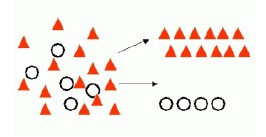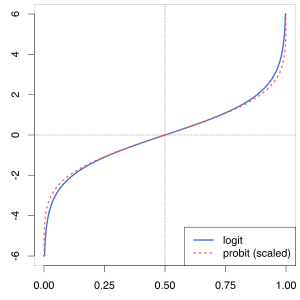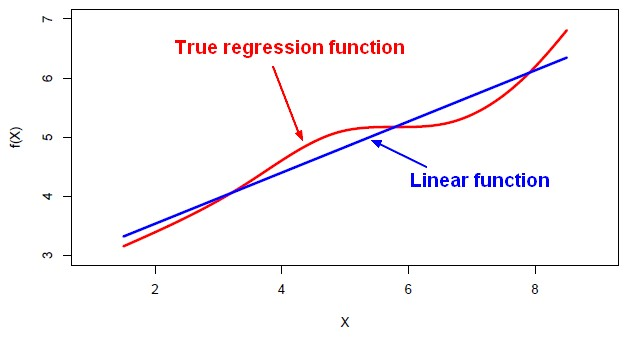# Data Mining - (two class|binary) classification problem (yes/no, false/true)

Binary classification is used to predict one of two possible outcomes.

A two class problem (binary problem) has possibly only two outcomes:

• “yes or no”
• “success” or “failure”

and is much more known as a Bernoulli trial (or binomial trial)

See

## Example

• Is this transaction a fraud ?
• Will this prospect become a customer ?
• Which employees are likely to leave a company in the next year
• Is the top card of a shuffled deck an ace?
• Was the newborn child a girl?
• Rolling a die, where a six is “success” and everything else a “failure”.
• In conducting a political opinion poll, choosing a voter at random to ascertain whether that voter will vote “yes” in an upcoming referendum.

Recommended Pages(Probability|Statistics) - Binomial Distribution

The binomial distribution is the discrete probability distribution of the number of successes in a sequence of n independent yes/no experiments, each of which yields success with probability p. The...Data Mining - (Classifier|Classification Function)

A classifier is a Supervised function (machine learning tool) where the learned (target) attribute is categorical (“nominal”) in order to classify. It is used after the learning process to classify...Data Mining - (Class|Category|Label) Target

A class is the category for a classifier which is given by the target. The number of class to be predicted define the classification problem. A class is also known as a label. Labeled...Data Mining - Probit Regression (probability on binary problem)

Probit_modelprobit model (probability + unit) is a type of regression where the dependent variable can only take two values. As the Probit function is really similar to the logit function, the probit...Data Mining - Problem

A page the problem definition in data Type of target: nominal or quantitative Type of target class: binomial of multiclass Number of parameters: Type of (predictor|features): nominal or numeric....Machine Learning - Area under the curve (AUC)

The Area under the curve (AUC) is a performance metrics for a binary classifiers. By comparing the ROC curves with the area under the curve, or AUC, it captures the extent to which the curve is up in the...Machine Learning - Linear (Regression|Model)

Linear regression is a regression method (ie mathematical technique for predicting numeric outcome) based on the resolution of linear equation. This is a classical statistical method dating back more...Statistics - (Confidence|likelihood) (Prediction probabilities|Probability classification)

Prediction probabilities are also known as: confidence (How confident can I be of this prediction?). or likelihood: (How likely is this prediction to be true?) They gives the probability of a predicted...Statistics - (Threshold|Cut-off) of binary classification

The Threshold or Cut-off represents in a binary classification the probability that the prediction is true. It represents the tradeoff between false positives and false negatives. Normally, the cut-off...Statistics - Binary logistic regression

logistic regression for a binary outcome. where: : predicted value on the outcome variable Y : the outcome variable : predicted value on Y when all X = 0 : predictor variables : unstandardized...